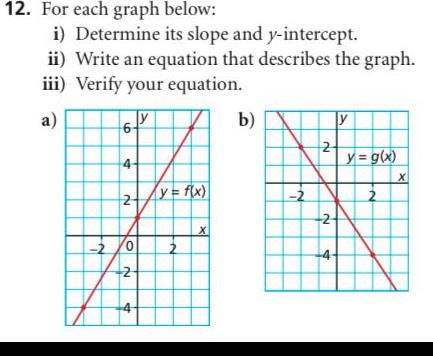Question:

# 12 For each graph below i Determine its slope and y

Last updated: 11/20/202312 For each graph below i Determine its slope and y intercept ii Write an equation that describes the graph iii Verify your equation a 6 4 2 2 0 2 N 4 y f x N X b 2 2 N 2 4 y y g x N X